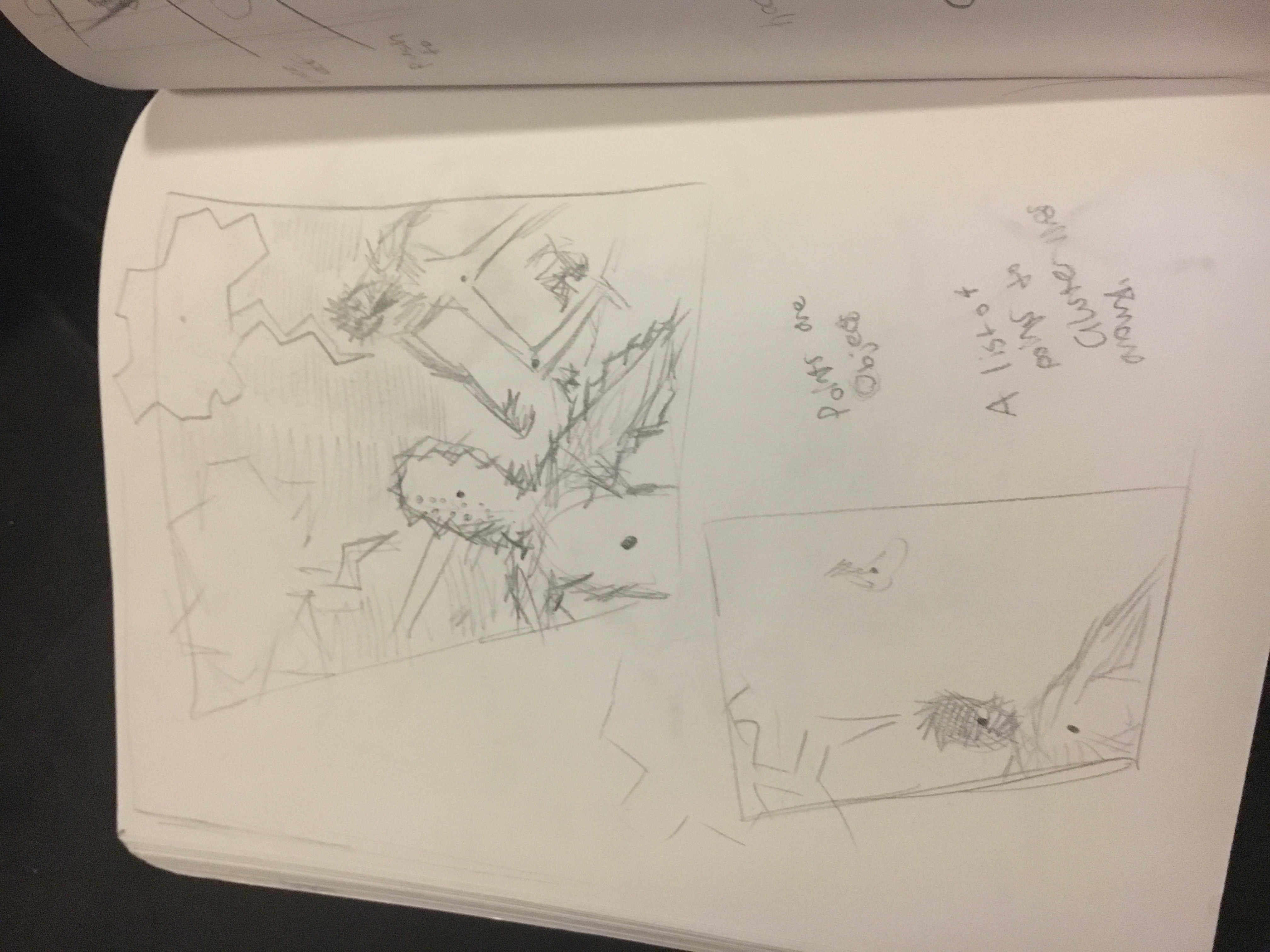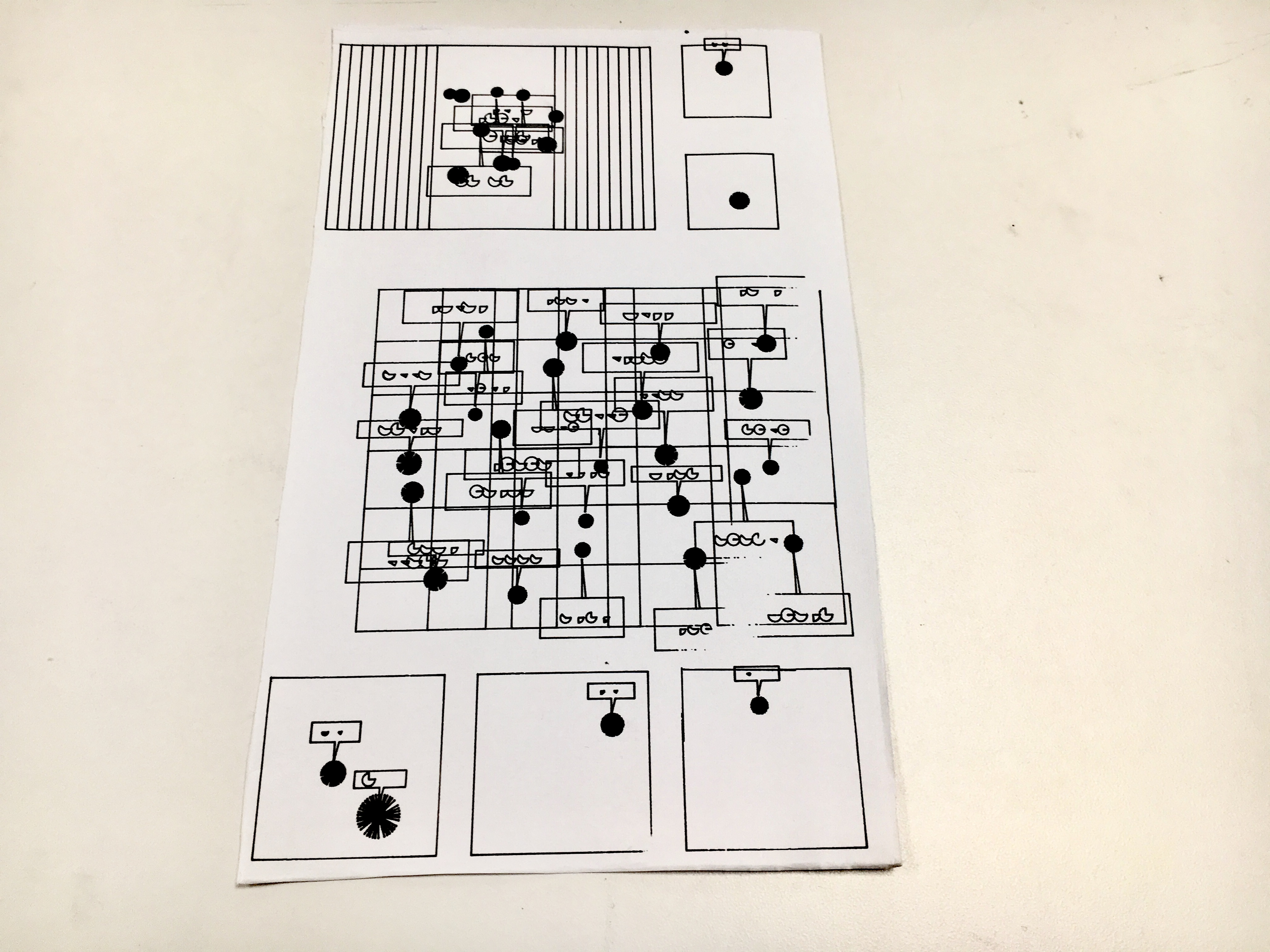# sheep – Asemic

sheep0The process of making the plot was pretty quick and dirty. I initially wanted to make something that involved sending an image into Processing and having processing turn all the black pixels into lines – it was going to be a comic. The comic format is still present, but instead of having representations I went for abstractions to make an “asemic comic.” The components of a comic are frames, speech bubbles, and characters. There is “dialogue” and “crowds.” The language for this piece wasn’t as important as the actual formatting. The plotter in action did not press down all the way, and I would have preferred to have a lot more time on it to work out the kinks. Poor planning on my part because of the overcrowding on the plotter was the greatest fault of this project. For the writing system itself, it’s just a bunch of random arcs, but I think they are evocative in that they remind me of mouths, so the aspect of speech is present- and the comic is entirely speech. I’m overall happy with the pdf, but my execution on the physical part could be better.

```  // see https://processing.org/reference/libraries/pdf/index.html import processing.pdf.*; boolean bRecordingPDF; int pdfOutputCount = 0; float border = 200; float frameBorder = 50; float radius = 100; float ghu; boolean grew; float spikes = 0; void setup() { size(900,1200); bRecordingPDF = true; }   void keyPressed() { // When you press a key, it will initiate a PDF export bRecordingPDF = true; }   void draw() { if (bRecordingPDF) { background(255); // this should come BEFORE beginRecord() beginRecord(PDF, "sheep" + pdfOutputCount + ".pdf"); rectMode(RADIUS); //-------------------------- noFill(); for (float i = 0; i &lt; 10; i++) { if (i%2 ==0) { grew = false; } else { grew = true; } panel pana1 = new panel(i *10 + 150, 150, grew); } for (float i = 0; i &lt; 5; i++) {   border = 50; frameBorder = 25; radius =50; for (float x = 0; x &lt; 5; x++) { grew = true; panel pana2 = new panel(i *(radius+5*i) + 150, x *radius+10 + 350, grew); } } ghu = 90;   radius = 40; panel pana3 = new panel(410, ghu, true); ghu += 120; panel pana4 = new panel(410, ghu, false); ghu += 500; radius = 65; spikes = 1; panel pana5 = new panel(105, ghu, true); spikes = 2; panel pana6 = new panel(260, ghu, true); spikes = 3; panel pana7 = new panel(415, ghu, true); //--------------------------   endRecord(); bRecordingPDF = false; pdfOutputCount++; } }   class chara { float x, y; float angle; float lineLength; chara (float xpos, float ypos, float l) { x = xpos; y = ypos; lineLength = l; } void update() { pushMatrix(); translate(x,y); rotate(random(360)); for (float i = 0; i &lt; 40; i++) { angle = i;   line(0, 0, cos(angle) * lineLength, sin(angle) * lineLength); } popMatrix(); } } class panel { panel(float a, float b, boolean tre) { float rx = a; float ry = b; rect(rx, ry, radius, radius); float ux = random(-radius+frameBorder, radius-frameBorder); float uy = random(-radius+frameBorder, radius-frameBorder); chara char1 = new chara(rx+ux, ry+uy, random(5, 10)); char1.update(); if (tre == true) { beginShape(); float angle = 180; float dist = random(30, 50) ; float distHeight = random(50, 70) ; float[] words = { QUARTER_PI, HALF_PI, (3*QUARTER_PI)/4, PI, PI+QUARTER_PI, PI + HALF_PI, PI + (3*QUARTER_PI)/4, TWO_PI, 0}; float multiplier = 10; if (char1.y &gt;ry ) {   dist = random(30, 50) ; distHeight = random(20, 70) ; multiplier = 10; } else {   dist = random(-50, -30) ; distHeight = random(-70, -50) ; multiplier = -10; } if (ghu &gt; 80) { dist = random (10, 20); distHeight = random(20, 30); multiplier = 10; } //vertex (char1.x + cos(angle)*10,char1.y + sin(angle)*10;   vertex (char1.x+ cos(angle), char1.y+ sin(angle)*multiplier); vertex (char1.x+ cos(angle) +3, char1.y+ sin(angle)*multiplier-distHeight/2); vertex ( char1.x+ cos(angle) + dist, char1.y+ sin(angle)*multiplier-distHeight/2); vertex (char1.x+ cos(angle)+dist, char1.y+ sin(angle)*multiplier -distHeight); vertex (char1.x+ cos(angle) -dist, char1.y+ sin(angle)*multiplier - distHeight); if (dist &gt; 0) { for (float d = 1; d&lt;dist; d +=10) {   arc((char1.x+ cos(angle) -((dist)/2))+d, char1.y+ sin(angle)*multiplier-((distHeight*3)/4), dist/4, dist/4, 0, words[int(random(words.length))], PIE); } } else if (dist &lt; 0) { for (float d = 1; d&gt;dist; d -=10) { arc((char1.x+ cos(angle) -((dist)/2))+d, char1.y+ sin(angle)*multiplier-((distHeight*3)/4), abs(dist)/4, abs(dist)/4, 0, words[int(random(words.length))], PIE); } } vertex (char1.x+ cos(angle) - dist, char1.y+ sin(angle)*multiplier - distHeight/2);   vertex(char1.x+ cos(angle), char1.y+ sin(angle)*multiplier - distHeight/2); vertex (char1.x+ cos(angle), char1.y+ sin(angle)*multiplier); endShape(); if (spikes == 1) { ux = random(-radius+frameBorder, radius-frameBorder); uy = random(-radius+frameBorder, radius-frameBorder); chara char2 = new chara(rx+ux, ry+uy, random(10, 20)); char2.update(); if (tre == true) { beginShape(); angle = 180; dist = random(30, 50) ; distHeight = random(50, 70) ;   multiplier = 10; if (char2.y &gt;ry ) {   dist = random(30, 50) ; distHeight = random(20, 70) ; multiplier = 10; } else {   dist = random(-50, -30) ; distHeight = random(-70, -50) ; multiplier = -10; } if (ghu &gt; 80) { dist = random (10, 20); distHeight = random(20, 30); multiplier = 10; } //vertex (char1.x + cos(angle)*10,char1.y + sin(angle)*10;   vertex (char2.x+ cos(angle), char2.y+ sin(angle)*multiplier); vertex (char2.x+ cos(angle) +3, char2.y+ sin(angle)*multiplier-distHeight/2); vertex ( char2.x+ cos(angle) + dist, char2.y+ sin(angle)*multiplier-distHeight/2); vertex (char2.x+ cos(angle)+dist, char2.y+ sin(angle)*multiplier -distHeight); vertex (char2.x+ cos(angle) -dist, char2.y+ sin(angle)*multiplier - distHeight); if (dist &gt; 0) { for (float d = 1; d&lt;dist; d +=10) {   arc((char2.x+ cos(angle) -((dist)/2))+d, char2.y+ sin(angle)*multiplier-((distHeight*3)/4), dist/2, dist/2, 0, words[int(random(words.length))], PIE); } } else if (dist &lt; 0) { for (float d = 1; d&gt;dist; d -=10) { arc((char2.x+ cos(angle) -((dist)/2))+d, char2.y+ sin(angle)*multiplier-((distHeight*3)/4), abs(dist)/2, abs(dist)/2, 0, words[int(random(words.length))], PIE); } } vertex (char2.x+ cos(angle) - dist, char2.y+ sin(angle)*multiplier - distHeight/2);   vertex(char2.x+ cos(angle), char2.y+ sin(angle)*multiplier - distHeight/2); vertex (char2.x+ cos(angle), char2.y+ sin(angle)*multiplier); endShape(); } } } } }```